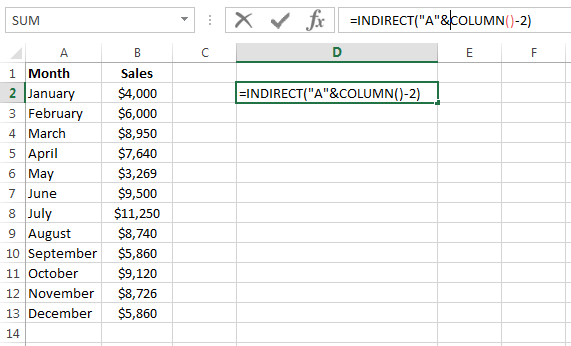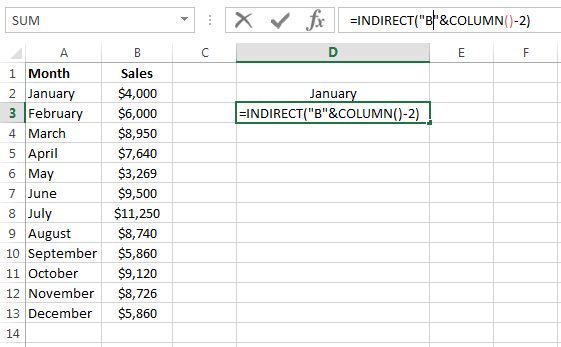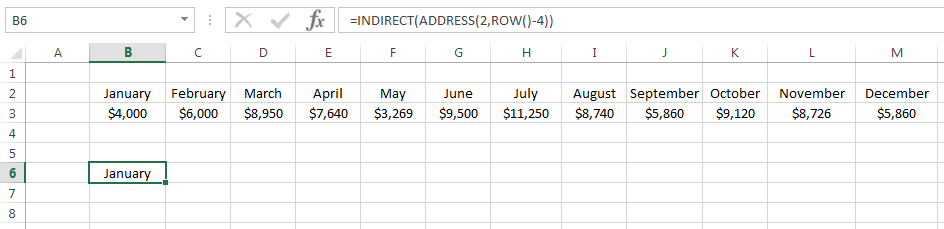Our Recent Posts

Archive

Tags

No tags yet.

# How to Transpose in Excel

### What is Transpose?

Transposing is making columns appear as rows and rows as columns.

You may have data which is arranged in columns but you want to re-arrange it to rows. I will show you how to transpose the data in Excel using two features:

1) Paste Special Transpose

2) Excel TRANSPOSE Function

3) Excel Indirect Function

### Paste Special TransposeI have a list of sales people across the columns with their sales from January to May. I want to transpose the sales people to the rows and the months along the columns.To transpose the data in Excel first copy it using the Ctrl+C shortcut keys.Click on the cell where you want to paste the transposed data then click on the Home tab on the ribbon. In the Clipboard group click on the down arrow on the Paste command button to bring a sub menu and then click on the Transpose button.The data is now transposed. You may want to delete the original data if it is not needed.

### Transpose Function

The TRANSPOSE function is another way to transpose data in Excel.

Transpose Argumentsarray – This is a required argument and is the range of cells that you want to transpose.

To Transpose the Data using the TRANSPOSE FunctionThe first thing to do is highlight the cell range where you want the data to be transposed to. Remember you want to transpose the rows to columns and vice versa. In this example in the data set there are 6 rows so I highlight 6 columns. There are 5 columns in the data set so I therefore highlight 5 rows so the cell range is H1:M5.In the top left cell, in this example cell H1, I type the formula =TRANSPOSE(A1:E6). The range argument is range A1:E6 in this example.This is an array formula so you need to press Control + Shift + Enter on your keyboard.

### TRANSPOSE Paste Special Values v TRANSPOSE Function

Which is the better method to transpose the data? The Transpose paste special values is definitely easier and quicker to do. However I prefer the TRANSPOSE function simply because if the source data changes then so does your transposed data. It is basically a dynamic transposed version of the source data.

### Using the Pivot Table to Transpose Data

Another quick and easy way to transpose data is by using a Pivot Table. You can very easily move the pivot items from the rows section to the columns section and vice versa.

### Using the INDIRECT Function to Transpose Data

Transpose Column to Row using INDIRECT FunctionIn this example there are sales figures for each month. I want to transpose this data from columns to rows.In cell D2 I enter the following formula =INDIRECT("A"&COLUMN()-2).In cell D3 I enter the formula =INDIRECT("A"&COLUMN()-2).I then use the fill handles to copy the formulas across.

Transpose Row to Column using INDIRECT FunctionIn this example I have the months and the sales figures across the columns and I want to transpose them in rows. This method is different to the previous example. You cannot just replace the COLUMN function with the ROW function. Instead you have to include the ADDRESS function with the INDIRECT and ROW function.In cell B6 I enter the following formula =INDIRECT(ADDRESS(2,ROW()-4)).In cell C6 I enter the formula =INDIRECT(ADDRESS(3,ROW()-4)).I then just copy the formulas down using the fill handles.

I hope you enjoyed this tutorial on how to transpose data in excel. If you have any questions or feedback please leave me a comment below.